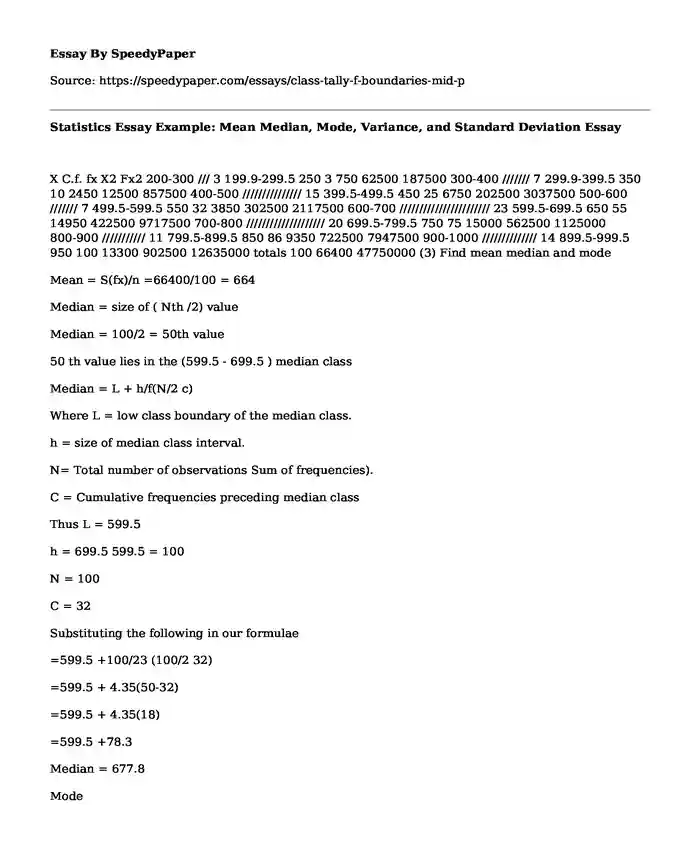# Statistics Essay Example: Mean Median, Mode, Variance, and Standard Deviation

Published: 2019-12-09Categories: Analysis Statistics Pages: 2 Wordcount: 496 words
143 views

X C.f. fx X2 Fx2 200-300 /// 3 199.9-299.5 250 3 750 62500 187500 300-400 /////// 7 299.9-399.5 350 10 2450 12500 857500 400-500 /////////////// 15 399.5-499.5 450 25 6750 202500 3037500 500-600 /////// 7 499.5-599.5 550 32 3850 302500 2117500 600-700 /////////////////////// 23 599.5-699.5 650 55 14950 422500 9717500 700-800 //////////////////// 20 699.5-799.5 750 75 15000 562500 1125000 800-900 /////////// 11 799.5-899.5 850 86 9350 722500 7947500 900-1000 ////////////// 14 899.5-999.5 950 100 13300 902500 12635000 totals 100 66400 47750000 (3) Find mean median and mode

Is your time best spent reading someone else’s essay? Get a 100% original essay FROM A CERTIFIED WRITER!

Mean = S(fx)/n =66400/100 = 664

Median = size of ( Nth /2) value

Median = 100/2 = 50th value

50 th value lies in the (599.5 - 699.5 ) median class

Median = L + h/f(N/2 c)

Where L = low class boundary of the median class.

h = size of median class interval.

N= Total number of observations Sum of frequencies).

C = Cumulative frequencies preceding median class

Thus L = 599.5

h = 699.5 599.5 = 100

N = 100

C = 32

Substituting the following in our formulae

=599.5 +100/23 (100/2 32)

=599.5 + 4.35(50-32)

=599.5 + 4.35(18)

=599.5 +78.3

Median = 677.8

Mode

Mode = L + (fm fm-1)/ {(fm fm-1) + (fm fm+1)}

L = low class boundary of the modal class.

Fm-1 = in the frequency of the group before the modal group

Fm = is the frequency of the modal group

Fm+1 = is the frequency of the group after modal class.

W =is the group with

L =599.5

fm-1 = 7

f m = 23

f m+1 =20

w = width of the modal class

substituting this in our formula we have

mode = 599.5 +{ (23-7) x}/ {(23 -7) (23 -20)}

mode = 599.9 + {(16/19) x 100 }

mode= 684.11

(4). Finding variance and standard deviation

Variance = S(X2/n) x2

Sx2 according top our frequency table = 47750000

N = 100

Mean = 664

Thus mean squared = 6642

= 440896

Substituting the following in the variance formulae

= {(47750000/100) 440896)

= 477500 440896

d2 = 36604

Standard deviation = variance

Standard deviation =36604

Standard deviation = 191.32

(5) .Compare mean, median, and state which you prefer as measure of location :From our data, our graph will portrays negative skew; the mean has the tendency of being pulled on the left side, which shows that the distribution is not symmetrical. Thus, median would be preferred as the measure of location of this data.

(6) .DRAW BAR GRAPH USING THE ABOVE:

(7). Find z value for smallest and largest data:

Z = (x-u)/d

X u ( X-u)

250 664 -414

350 664 -314

450 664 -214

550 664 -114

650 664 -14

750 664 86

850 664 186

950 664 286

Replacing the values in our formulae

Standard deviation = 191.32 as calculated earlier

Z = - 414/191.32

Z = -2.19

Z = 286/191.32 = 1.49

2.16 < z < 1.49

Statistics Essay Example: Mean Median, Mode, Variance, and Standard Deviation. (2019, Dec 09). Retrieved from https://speedypaper.com/essays/class-tally-f-boundaries-mid-p

Request Removal

If you are the original author of this essay and no longer wish to have it published on the SpeedyPaper website, please click below to request its removal:

Liked this essay sample but need an original one?

Hire a professional with VAST experience!